# SAT Math: Geometry and Measurement Chapter Exam

Exam Instructions:

Choose your answers to the questions and click 'Next' to see the next set of questions. You can skip questions if you would like and come back to them later with the yellow "Go To First Skipped Question" button. When you have completed the practice exam, a green submit button will appear. Click it to see your results. Good luck!

### Page 1

#### Question 2 2. Triangle ABC is equilateral. If line BD bisects angle ABC, and the length of BD is 3√3, what is the perimeter of Triangle ABC?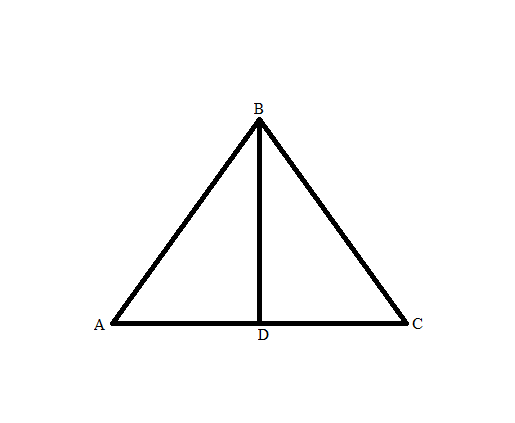#### Question 4 4. What is the curve length of f(x) between x=0 and x=1?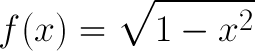#### Question 5 5. Which two lines are parallel?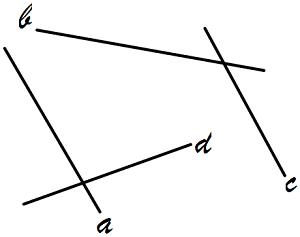### Page 2

#### Question 6 6. In the square ABCD shown below, the measure of angle BCA is equivalent to the measure of ACD, and the measure of angle ACE is equivalent to the measure of angle ECD. What is the measure of angle CED?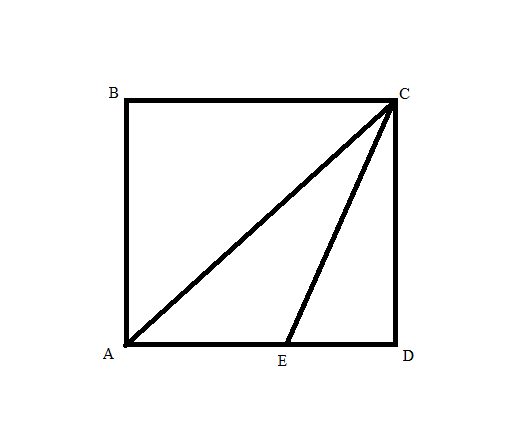#### Question 7 7. In the figure below, Angle 7 = 15x and Angle 10 = 9x + 30. Determine the measure of Angle 10.#### Question 8 8. Which theorem can be used to establish congruence with the pictured triangles?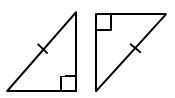### Page 3

#### Question 12 12. What is the area of the pictured triangle?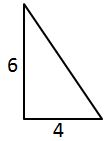#### Question 13 13. What is the area of ABCDE, as shown in the diagram?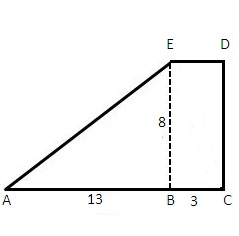#### Question 14 14. In triangle GBN, Angle G is seven more than three times a number, Angle B is five more than four times the number, and Angle N is twelve less than five times the number. Solve for the measure of Angle G.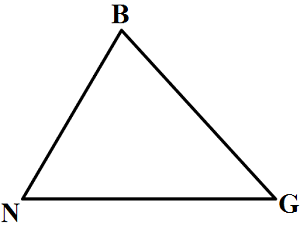### Page 4

#### Question 16 16. In the picture below, if AB is 12, AC is 24 and AD is 10, what is the length of AE?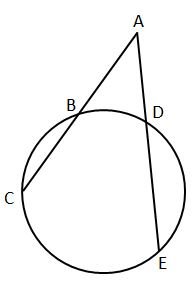#### Question 17 17. A loading ramp is to be stretched from the back of a truck down to the ground, as shown. If the length of the ramp is 25 feet, and the vertical distance covered by the ramp is 15 feet, then how far away from the truck does the ramp touch the ground?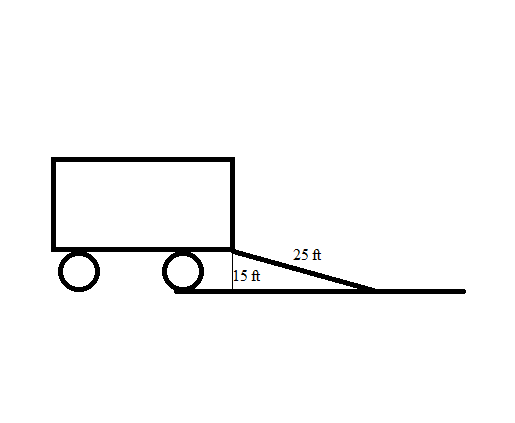#### Question 18 18. What is the equation for the length of the curve following y=f(x) between x=a and x=b given f(x) below?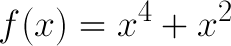#### Question 19 19. Line CD is parallel to Line EF. If the measure of angle FGA is one-half the measure of angle EGA, then what is the measure of angle DHB?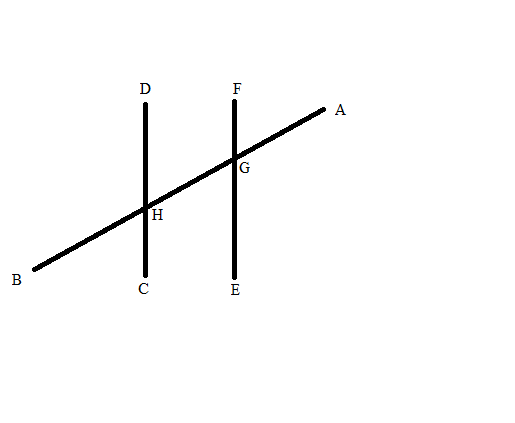### Page 5

#### Question 23 23. If arc AB is 72 degrees, what is the measure of angle ACB?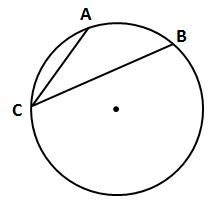#### Question 25 25. What is the line, as shown in the picture, called?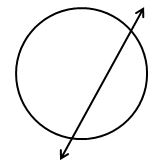### Page 6

#### Question 28 28. A cube is 4 inches on a side, as shown in the image. What is the diameter, in inches, of the smallest sphere that will contain the cube?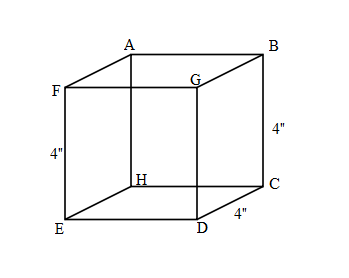#### Question 30 30. Which theorem can be used to establish congruence with the pictured triangles?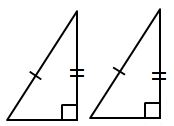#### SAT Math: Geometry and Measurement Chapter Exam Instructions

Choose your answers to the questions and click 'Next' to see the next set of questions. You can skip questions if you would like and come back to them later with the yellow "Go To First Skipped Question" button. When you have completed the practice exam, a green submit button will appear. Click it to see your results. Good luck!

Support